Question

# What is the pH of a solution containing 0.831 mol L-1 of a diprotic acid with...

What is the pH of a solution containing 0.831 mol L-1 of a diprotic acid with pKA1 = 4.62 and pKA2 = 8.62 ?

H2A + H2O ⇌ H3O+ + HA- pkA1

HA- + H2O ⇌ H3O+ + A2- pkA2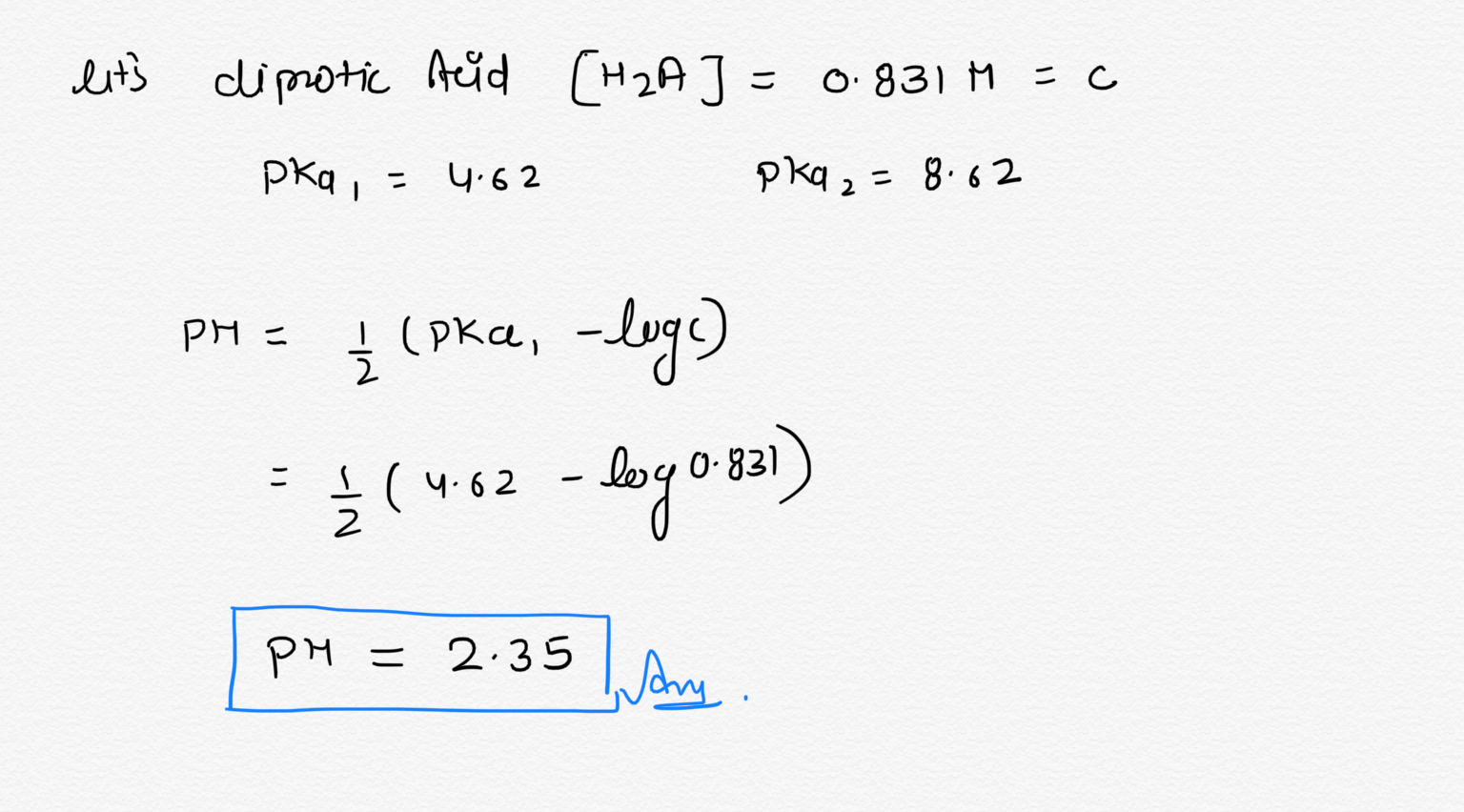#### Earn Coins

Coins can be redeemed for fabulous gifts.

Similar Homework Help Questions
• ### Consider the acid dissociation reactions and for the diprotic acid H2A: H2A(aq) + H2O (l) --><--...

Consider the acid dissociation reactions and for the diprotic acid H2A: H2A(aq) + H2O (l) --><-- HA-(aq) + H3O+(aq) pKa1= 3 H2A(aq) + H2O (l) --><-- A2- (aq) + H3O+(aq) pKa2= 8 Would a salt solution of KHA be acidic, basic or neutral? a) Acidic b) Basic c) Neural

• ### 4. A diprotic acid has the following pK values: pKa1 = 5.50, pKa2 = 9.50 A....

4. A diprotic acid has the following pK values: pKa1 = 5.50, pKa2 = 9.50 A. At what pH does [H2A] = [HA- ]? B. At what pH doe [HA- ] = [A2- ]? C. Which is the principal species at pH = 3.50, H2A, HA- , or A2- ? Justify your answer using the Henderson-Hasselbach equation. D. Which is the principal species at pH = 7.5, H2A, HA- , or A2- ? Justify your answer (don’t use Henderson-Hasselbach equation,...

• ### Malonic acid [ molecular formula C3H4O4] is a diprotic acid with pKa1 = 2.83 and pKa2...

Malonic acid [ molecular formula C3H4O4] is a diprotic acid with pKa1 = 2.83 and pKa2 = 5.69 . Determine the concentrations of the neutral acid, the monoanion and the dianion [H2A, HA- and A2-] in a solution contaning 50.0 mg of malonic acid in 1.00 liter of solution.

• ### Part E A diprotic acid has a pKa1 -2.80 and pka2 6.50. What is the pH of a 0.10 M solution of thi...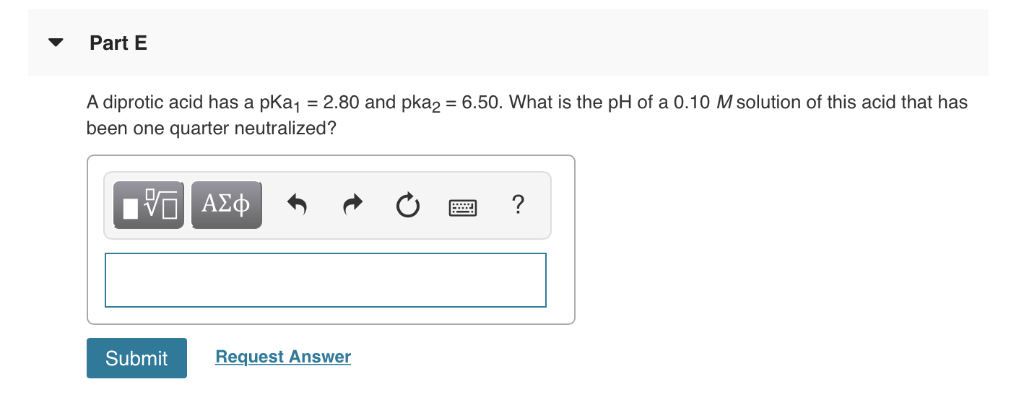Part E A diprotic acid has a pKa1 -2.80 and pka2 6.50. What is the pH of a 0.10 M solution of this acid that has been one quarter neutralized? 97 ΑΣΦ Submit Request Answer Part E A diprotic acid has a pKa1 -2.80 and pka2 6.50. What is the pH of a 0.10 M solution of this acid that has been one quarter neutralized? 97 ΑΣΦ Submit Request Answer

• ### Calculate the pH at each point listed for the titration of 100.00mL of 0.100M Diprotic acid...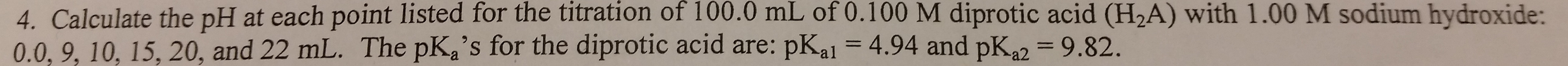Calculate the pH at each point listed for the titration of 100.00mL of 0.100M Diprotic acid (H2A) with 1.00M Sodium hydroxide: 0.0, 9, 10, 15, 20, and 22mL. The pKa's for the diprotic acid are: pKa1=4.94 and pKa2=9.82. Please show any work, a photo of a solution would be preferred but not necessary...Thanks for any help!

• ### A diprotic acid, H2A has acid dissociation values, with pKa1-1.85 and pKa2-7.17. What is the Kb1...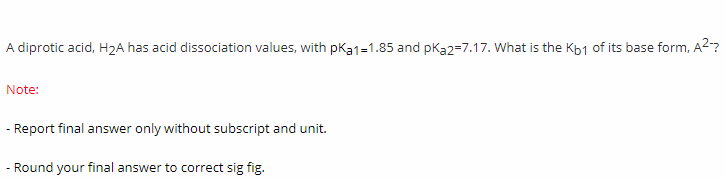A diprotic acid, H2A has acid dissociation values, with pKa1-1.85 and pKa2-7.17. What is the Kb1 of its base form, A2? Note: -Report final answer only without subscript and unit. - Round your final answer to correct sig fig.

• ### For the diprotic weak acid H2A, ?a1=2.0×10−6 and ?a2=8.8×10−9. What is the pH of a 0.0650 M solution of H2A? pH= What ar...

For the diprotic weak acid H2A, ?a1=2.0×10−6 and ?a2=8.8×10−9. What is the pH of a 0.0650 M solution of H2A? pH= What are the equilibrium concentrations of H2A and A2− in this solution? [H2A]= M[A2−]=

• ### home / study / science / chemistry / chemistry questions and answers / a diprotic acid...

home / study / science / chemistry / chemistry questions and answers / a diprotic acid has a pka1 = 2.90﻿ and pka2 = 6.50. what is the ph of a 0.10 m solution of ... Question: A diprotic acid has a pKa1 = 2.90﻿ and pka2 = 6.50. What is the pH of a 0.10 M solution of this a... A diprotic acid has a pKa1 = 2.90﻿ and pka2 = 6.50. What is the pH of a 0.10...

• ### Titration of a diprotic acid with a strong base You have a 10.0 mL solution containing...

Titration of a diprotic acid with a strong base You have a 10.0 mL solution containing 0.5 M carbonic acid. Carbonic acid is diprotic, with pKa1 = 6.35 and pKa2 = 10.33. You titrate this solution using 1.00 M NaOH . (a) Calculate the pH of the solution before adding any NaOH. (b) Calculate the amount of NaOH needed to reach the first midpoint. What is the pH? (c) Calculate the amount of NaOH needed to reach the first equivalence...

• ### A diprotic acid, H2A, has acid dissociation constants of Ka1=1.01×10−4 and Ka2=4.08×10−12. Calculate the pH and...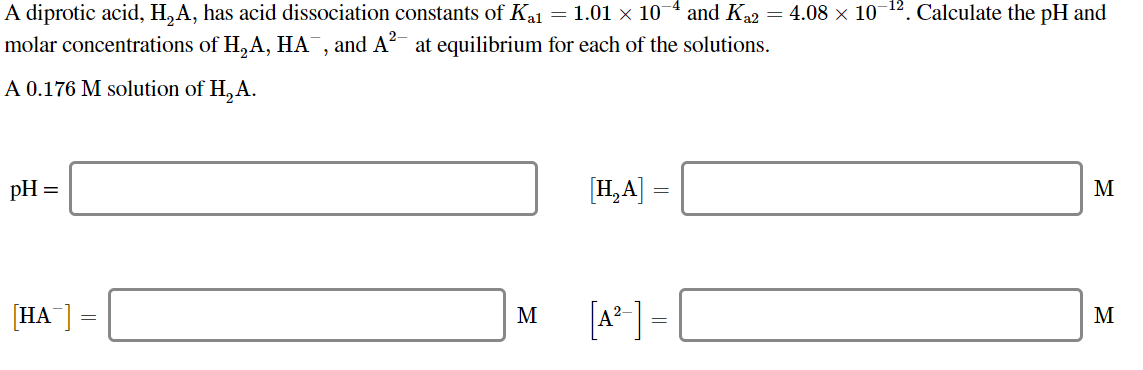A diprotic acid, H2A, has acid dissociation constants of Ka1=1.01×10−4 and Ka2=4.08×10−12. Calculate the pH and molar concentrations of H2A, HA−, and A2−at equilibrium for each of the solutions. A diprotic acid, H, A, has acid dissociation constants of Kal = 1.01 x 104 and K22 = 4.08 x 10-12. Calculate the pH and molar concentrations of H, A, HA, and A? at equilibrium for each of the solutions. A 0.176 M solution of H, A. pH= pH = 1...